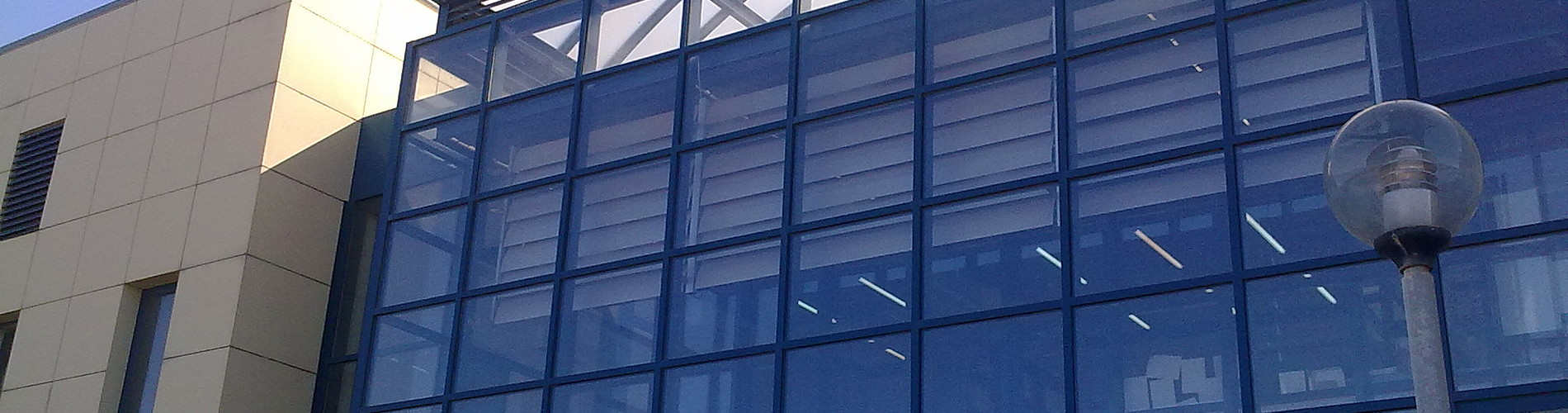School of Chemical and Environmental Engineering

Now offering two distinct diplomas: Chemical Engineering and Environmental Engineering

# Differential and Integral Calculus II

1. COURSE INFORMATION:

 School Chemical and Environmental Engineering Course Level Undergraduate Direction - Course ID MATH 102 Semester 2nd Course Category Required Course Modules Instruction Hours per Week ECTS Lectures and Tutorial Exercises 4 Th=4, E=1, L=0 4 Course Type General Background Prerequisites Instruction/Exam Language Greek The course is offered to Erasmus students No Course URL https//www.eclass.tuc.gr/courses/MHPER295   (in Greek)

2. LEARNING OUTCOMES

 Learning Outcomes The goal of the class Infinitesimal Calculus ΙI is to provide the basic tools of mathematics needed by engineers in the theoretical part and the calculating part of his/her education.With the successful completeness of the course the student should to know to:Execute calculations with vectors.Analyze a  force to components and find its projection to any direction.Calculate areas of parallelograms and volumes of parallelepipeds obtained with the help of vectors.Present equations of lines and planes in the space.Differentiate functions and find partial derivatives of functions of two or three variables.Calculate the partial derivatives of implicit functions given by equation.Know to use the directional derivative and the gradient of a function and be able to determine when a vector field is the gradient of a function.Find the extreme points of functions of 2 variables on an open or closed set with the criterion of second derivative. Calculate volumes of solids with double or triple integrals. Calculate areas of surfaces, the work of a force along a curve in the space.Determine whether a vector field is conservative.Calculate the flow of a liquid along a plane curve.Use the Div and the Curl of a vector field and know the relation of these notions with the flow of the vector field through a curve or a surface (theorems of Green, Stokes, Gauss).evaluate the divergence and rotation of a vector field and their relation with the flow  of fluid  across  along or across a plane curve (Green’s theorem), the boundary of a surface (Stoke’s theorem) or along a closed surface (Gauss theorem). General Competencies/Skills Search, analysis and synthesis of data and information.Independent work.Team work.Work in scientific environment.Produce free deductive thinking.

3. COURSE SYLLABUS

 Basic vector algebra. Inner, cross, triple product of vectors.Equations of lines, and planes in the space.Polar, cylindrical, spherical coordinates.Curves. Derivative and integral of vector functions.Speed, acceleration.Functions of two or more variables. Partial Derivatives of Multivariable Functions.Chain rule.Directional Derivative.Functions of two or more variables. Gradient vector and tangent planes.Extrema of functions of several variables.Lagrange Multipliers.Line integrals.Work of force, Conservative fields.Double, triple integrals.Applications in Physics and Geometry (volumes of solids, moments of inertia).The Green Theorem. Flow of vector field along or across a curve.Surface Integrals.Parametric representation of surfaces and applications in the flow of fluids.The Stokes Theorem. Gauss's Divergence Theorem.

4. INSTRUCTION and LEARNING METHODS - ASSESSMENT

 Lecture Method Direct (face to face) Use of Information and Communication Technology E-class support Instruction Organisation Activity Workload per Semester (hours) - Lectures 52 - Tutorial Exercises 13 - Autonomous study 35 Course Total 100 Assessment Method Ι. Written final examination (100%)